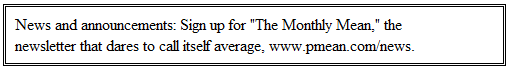P.Mean: Putting variable names into a model automatically (created 2010-09-20, updated 2011-12-02).I always have trouble with including a changing variable name into a sequence of statistical models in R, so when someone wrote about it on the R-Help list, I thought I should try some of the suggestions and then write them down here so I don't forget.

Here's the problem. If you run models inside a loop, the information about models is not properly documented as part of the output. There is a small data set on house prices that can help illustrate this problem. Here are the first five rows of the data set.

```> housing[1:5,]   sqft age feats nec cust cor  price 1 2650  13     7   1    1   0 205000 2 2600  NA     4   1    1   0 208000 3 2664   6     5   1    1   0 215000 4 2921   3     6   1    1   0 215000 5 2580   4     4   1    1   0 199900 ```

The first three variables are the square footage, age, and the number of special features of the house. The next three variables are indicators for whether the house is in the northeast corner of the city, whether it is a custom built house, and whether it is on a corner lot. The last variable is the sales price of the house. You might be interested in what variables predict sales price. Part of the preliminary analysis would be to look at simple univariate regression before fitting a more complex model. You could do this inside a loop, but when you do, the information about the individual variables is not carried along with the output. For example, this simple loop

`for (i in 1:6) {print(coef(lm(price~housing[,i])))}`

produces the following:

``` (Intercept) housing[, i]   4781.93066     61.36668  (Intercept) housing[, i] 116847.00710    -24.75735  (Intercept) housing[, i]     66117.42     11375.94  (Intercept) housing[, i]     97282.05     13487.18  (Intercept) housing[, i]     94752.22     49925.56  (Intercept) housing[, i]   107718.947    -7687.129```

The same problem occurs if you work with a function:

```univ.coef <- function(dv,iv) {coef(lm(dv~iv))} univ.coef(price,sqft)```

which produces the following output

```(Intercept)      iv 4781.93066 61.36668```

Inside a loop, you can paste the model together.

```for (i in 1:6) {  tmp.formula <- as.formula(paste("price ~",names(housing)[i]))  print(coef(lm(tmp.formula))) `````` }```

which produces the following output

``` (Intercept)      sqft  4781.93066   61.36668  (Intercept)       age 116847.00710 -24.75735  (Intercept)     feats     66117.42  11375.94  (Intercept)       nec     97282.05  13487.18  (Intercept)      cust     94752.22  49925.56  (Intercept)       cor   107718.947 -7687.129```

This also works within a function, but you have to place your variable names in quotes.

```univ.coef <- function(dvname,ivname) {  tmp.formula <- as.formula(paste(dvname,"~",ivname))  coef(lm(tmp.formula)) } univ.coef("price","sqft")```

which produces the following output

``` (Intercept)      sqft   4781.93066  61.36668```

There was a similar question about functions. Suppose you have a character vector that represented the name of a function. You could convert that string to the actual function with the match.fun function. For example,

```test.data <- 1:10 flist <- c("min","mean","max") stat <- numeric(0) for (i in 1:length(flist)) {  fun <- match.fun(flist[i])  stat[i] <- fun(test.data) } stat```

produces the following output

`  1.0 5.5 10.0`

Another more complicated approach is

```test.data <- 1:10 flist <- c("min","mean","max") stat <- numeric(0) for (i in 1:length(flist)) {  stat[i] <- eval(as.call(list(as.name(flist[i]), test.data))) } stat```

which produces the following output

`  1.0 5.5 10.0`

There's nothing wrong, though, with storing the functions themselves

```test.data <- 1:10 flist <- c(min,mean,max) stat <- numeric(0) for (i in 1:length(flist)) {  stat[i] <- flist[[i]](test.data) }```
`stat`

which produces the following output

`  1.0 5.5 10.0`

You have to use double brackets because functions are combined as a list, not as a vector.

You might ask yourself, why go to all this trouble. One advantage of incorporating variables names from a list into a model or converting the string name of a function into the function itself is to that you can use the same string to create dimension names in a matrix, or incorporate those strings into the title of a graph. It also allows you to dynamically change the function list within the program.This work is licensed under a Creative Commons Attribution 3.0 United States License. This page was written by Steve Simon and was last modified on 2017-06-15. Need more information? I have a page with general help resources. You can also browse for pages similar to this one at Category: R software.